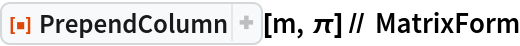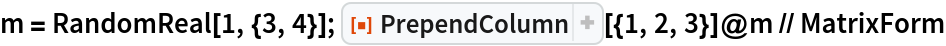Function Repository Resource:

# PrependColumn

Prepend a column to the left of a matrix

Contributed by: Michael Sollami
 ResourceFunction["PrependColumn"][matrix,list] prepends a vector list as a column to matrix. ResourceFunction["PrependColumn"][matrix,s] prepends a constant vector of scalar s to matrix. ResourceFunction["PrependColumn"][column] represents an operator form of PrependColumn that can be applied to a matrix.

## Details and Options

Dimensions of matrix and list must be compatible.

## Examples

### Basic Examples (2)

Prepend a column vector to a matrix:

 In:=Out=Append a scalar value to a matrix:

 In:=Out=### Scope (1)

Use PrependColumn as an operator form:

 In:=Out=Michael Sollami

## Version History

• 1.0.0 – 23 March 2020